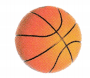# Texas Go Math Grade 2 Lesson 15.1 Answer Key Three-Dimensional Solids

Refer to our Texas Go Math Grade 2 Answer Key Pdf to score good marks in the exams. Test yourself by practicing the problems from Texas Go Math Grade 2 Lesson 15.1 Answer Key Three-Dimensional Solids.

## Texas Go Math Grade 2 Lesson 15.1 Answer Key Three-Dimensional Solids

Explore

Draw a picture of an object that has the shape shown.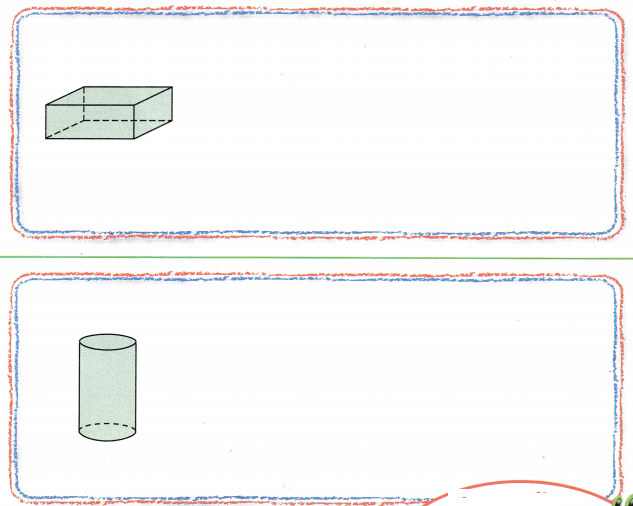FOR THE TEACHER • Have children look at the first shape and name some real objects that have this shape, such as a cereal box. Have each child draw a picture of a real-life object that has the same shape. Repeat for the second shape.

Math Talk
Mathematical Processes

Describe how the shapes are alike. Describe how they are different.

Model and Draw

These are three-dimensional solids. Compare the shapes and surfaces of the different solids.Which of these objects has the shape of a cube?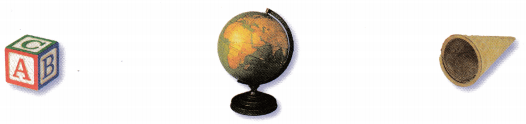Explanation:
In the given objects, the ABC box  resembles the cubeShare and Show

Circle the objects that match the name of the solid.

Question 1.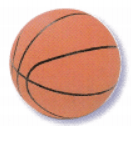Question 2.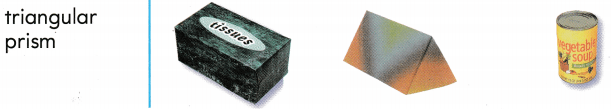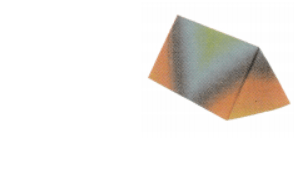Problem Solving

Circle the objects that match the name of the solid.

Question 3.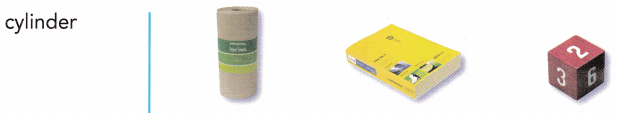Question 4.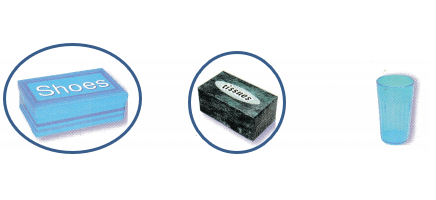Explanation:
Both the images resemble a rectangular prism

Question 5.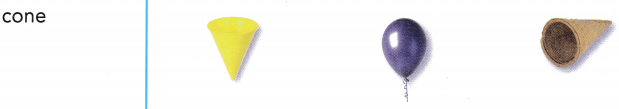Explanation:
Both the images resemble cones.

Question 6.
H.O.T. Multi-Step Circle the solids that have a curved surface. Draw an X on the solids that do not have a curved surface.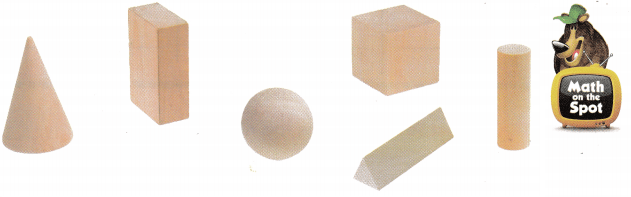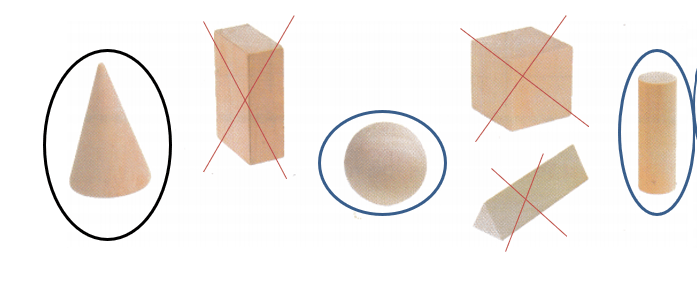Question 7.
H.O.T. Julio used cardboard squares as the flat surfaces of a cube. How many squares did he use?
_____________ squares
8 squares

Explanation:
Julio used cardboard in the shape of a cube, which has squares on all sides. So there are 6 squares on the cardboard.Write the shape names to complete.Question 8.
Choose the best label for each solid. Then write the names of the solids.
Has only flat surfaces

Has only a curved surface

Has both flat and curved surfaces
Has only flat surfaces:Has only a curved surface:Has both flat and curved surfaces: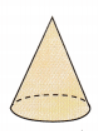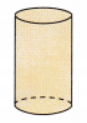Question 9.
Texas Test Prep What is the name of this solid?(A) triangular prism
(B) cone
(C) cylinder
Cone

TAKE HOME ACTIVITY • Ask your child to name on object that has the shape of a cube.### Texas Go Math Grade 2 Lesson 15.1 Homework and Practice Answer Key

Circle the objects that match the name of the solid.

Question 1.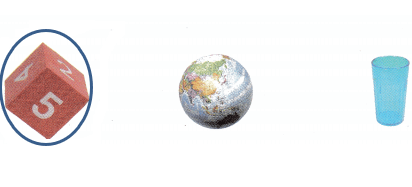Question 2.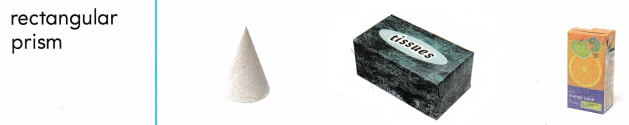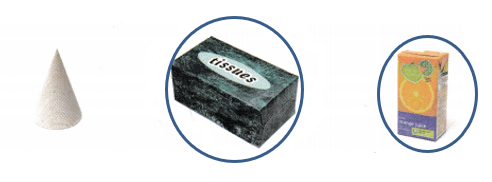Question 3.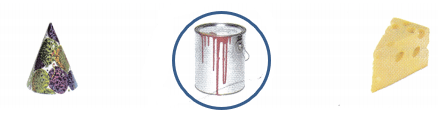Problem Solving

Question 4.
Multi-Step Circle the objects that have a curved surface. Draw an X on the objects that do not have a curved surface.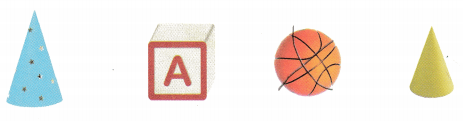Lesson Check

Question 5.
Which of these objects is shaped like a cylinder?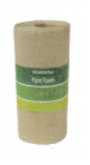Question 6.
James sees this box. Which solid names the shape of this box?(A) cone
(B) triangular prism
(C) cylinder
Triangular prism

Question 7.
Kara loves to wrap gift boxes. Which solid names the shape of her gift box?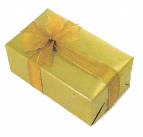(A) rectangular prism
(B) triangular prism
(C) sphere